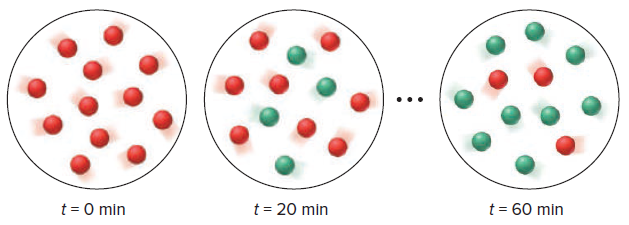# Problem: The molecular scenes below represent the first-order reaction in which cyclopropane (red) is converted to propene (green):Determine (b) the rate constant.

###### FREE Expert Solution
82% (108 ratings)
###### Problem Details

The molecular scenes below represent the first-order reaction in which cyclopropane (red) is converted to propene (green):Determine (b) the rate constant.

Frequently Asked Questions

What scientific concept do you need to know in order to solve this problem?

Our tutors have indicated that to solve this problem you will need to apply the Integrated Rate Law concept. You can view video lessons to learn Integrated Rate Law. Or if you need more Integrated Rate Law practice, you can also practice Integrated Rate Law practice problems.

What textbook is this problem found in?

Our data indicates that this problem or a close variation was asked in Chemistry: The Molecular Nature of Matter and Change - Silberberg 8th Edition. You can also practice Chemistry: The Molecular Nature of Matter and Change - Silberberg 8th Edition practice problems.